# Cubic hypersurface

(diff) ← Older revision | Latest revision (diff) | Newer revision → (diff)

A projective algebraic variety defined by a homogeneous equation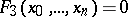of degree three with coefficients in some ground field.

## Cubic curves.

An irreducible cubic curve is either smooth (in which case its canonical class is 0, its genus 1) or has a unique singular double point (in which case it is rational). Cubic curves are the curves of lowest degree for which there exist moduli (cf. Moduli theory). Every smooth cubic curveover an algebraically closed fieldof characteristic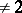or 3 can be reduced by birational transformations to Weierstrass form, which is, in terms of non-homogeneous coordinates on the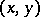-plane,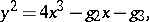where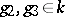,. Two cubic curves with coefficientsand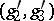in Weierstrass form are isomorphic if and only if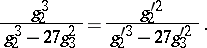The function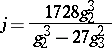takes arbitrary values inand depends only on the curve; it is called the absolute invariant of.

One can define a binary composition law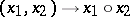on the set of points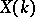of a cubic curve: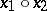is the third point of intersection ofwith the straight line through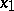and. If one fixes some point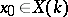, the compositionturnsinto an Abelian group with neutral element. A cubic curve endowed with this structure is a one-dimensional Abelian variety (an elliptic curve).

If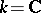is the field of complex numbers,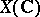is a Riemann surface of genus 1, i.e. a one-dimensional complex torus — a quotient group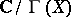, whereis a two-dimensional period lattice. The fieldof rational functions of the curveis then isomorphic to the field of elliptic functions onwith period lattice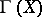. The coefficients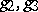are interpreted as modular forms of weight 4 and 6, respectively, that are identical, up to a constant factor, with the forms defined by Eisenstein series of lowest weights. In that case the functionis none other than the modular invariant.

A rich arithmetic theory has also been developed for cubic curves over algebraically non-closed fields(see ). Significant achievements in that respect are the Mordell–Weil theorem, the theory of complex multiplication and the homology theory of principal homogeneous spaces. The main unsolved problems (as of 1982) are: boundedness of the rank over an algebraic number field; the finiteness conjecture for the group of principal homogeneous locally trivial spaces; the conjecture of Birch and Swinnerton-Dyer on the zeta-function; Weil's uniformization conjecture, etc. (See also Elliptic curve.)

## Cubic surfaces.

Over an algebraically closed field, every irreducible cubic surface (that does not degenerate into a cone) is a rational surface. The class of a hyperplane sectionof a surfaceis precisely the canonical class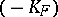. Any smooth cubic surface can be obtained from the projective plane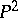by blowing-up (i.e. performing a monoidal transformation) of 6 points, no three of which are collinear, which do not lie on a single conic. The appropriate birational mapping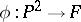is determined by the linear system of cubic curves passing through the 6 points. There are 27 straight lines on, each of which is exceptional (see Exceptional subvariety); they are the only exceptional curves on. The configuration of these 27 lines is rich in symmetries: The automorphism group of the corresponding graph is isomorphic to the Weil group of type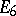. Cubic surfaces belong to the class of del Pezzo surfaces — projective surfaces with an ample anti-canonical class.

Over an algebraically non-closed field, there are smooth cubic surfaceswhich are not birationally isomorphic toover(i.e.is not rational over). Among these surfaces one finds surfaces possessing-points, and these are unirational over. Such cubic surfaces provide a counterexample for the Lüroth problem on surfaces over non-closed fields. There exist fieldsover which there are minimal cubic surfaces. Segre's minimality criterion :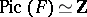. The group of birational automorphisms of a minimal surface has been determined (in terms of its generators and defining relations) and an arithmetic theory of cubic surfaces has been developed . In order to describe the set of points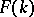one appeals to non-associative structures, such as quasi-groups and Moufang loops.

## Cubic hypersurfaces of dimension 3.

All smooth cubic hypersurfaces of dimensionover an algebraically closed field are unirational. As far back as the eighties of the 19th century, the following question was posed: Is a smooth three-dimensional cubic hypersurface rational? A negative answer has been obtained . This also provides a negative solution to the Lüroth problem for three-dimensional varieties. For every smooth three-dimensional cubic hypersurfacethere exists a principal polarized five-dimensional Abelian variety — the intermediate Jacobian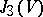. Ifit is defined as as the complex toruswhere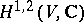is the corresponding Hodge component in the decomposition of the homology space. In order to prove thatis non-rational, it was shown thatis not the Jacobian of any curve of genus 5. The fact that a cubic hypersurface over a field of finite characteristic is non-rational was established in .

A cubic hypersurfaceis uniquely determined by its Fano surface. For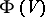one has the Torelli theorems (which are also valid foritself). The following problem is unsolved: Given a three-dimensional cubic hypersurface, describe its group of birational automorphisms.

It is not known (1987) whether every smooth cubic hypersurface of dimension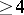is rational. Rationality has been proved in this case for certain hypersurfaces of a special type; for example: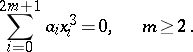How to Cite This Entry:
Cubic hypersurface. Encyclopedia of Mathematics. URL: http://encyclopediaofmath.org/index.php?title=Cubic_hypersurface&oldid=17877
This article was adapted from an original article by V.A. Iskovskikh (originator), which appeared in Encyclopedia of Mathematics - ISBN 1402006098. See original article